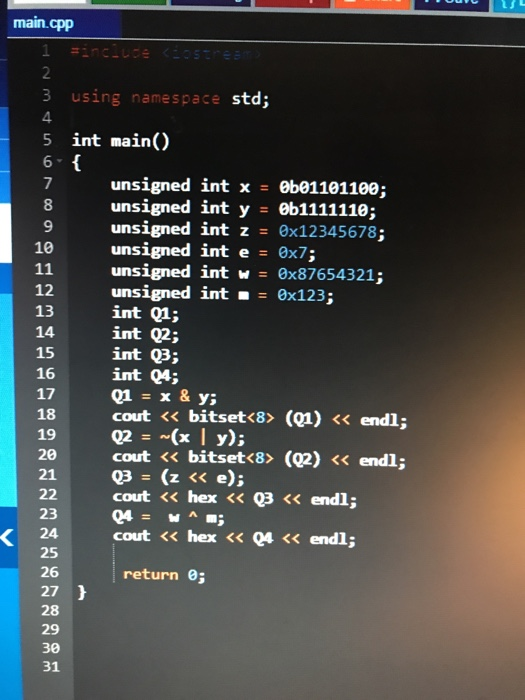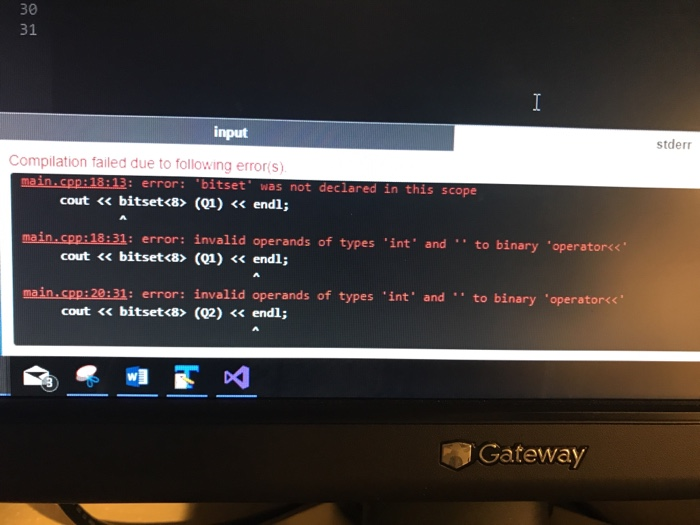# Question & Answer: main.cpp 2 3 using namespace 4. 5 int main() std; unsigned int x = 0b01101100; 8 9 10 12 13 14 15 16 17 18 19 20 21 unsigned int z = 0x123…..

How can I fix that ? Can you please explain clearly ?main.cpp 2 3 using namespace 4. 5 int main() std; unsigned int x = 0b01101100; 8 9 10 12 13 14 15 16 17 18 19 20 21 unsigned int z = 0x12345678; unsigned int e 0x7; unsigned int w = 0x87654321; unsigned int =0x123; int 1; int 92; int Q3; int Q4; Q1=x&y; cout

#include <iostream>
#include <bitset>
using namespace std;

Don't use plagiarized sources. Get Your Custom Essay on
Question & Answer: main.cpp 2 3 using namespace 4. 5 int main() std; unsigned int x = 0b01101100; 8 9 10 12 13 14 15 16 17 18 19 20 21 unsigned int z = 0x123…..
GET AN ESSAY WRITTEN FOR YOU FROM AS LOW AS \$13/PAGE

int main()
{
unsigned int x = 0b01101100;
unsigned int y = 0b1111110;
unsigned int z = 0x12345678;
unsigned int e = 0x7;
unsigned int w = 0x87654321;
unsigned int m = 0x123;

int Q1, Q2, Q3, Q4;

Q1 = x & y;
cout << bitset<8> (Q1) << endl;
Q2 = ~(x | y);
cout << bitset<8> (Q2) << endl;
Q3 = (z << e);
cout << hex << (Q2) << endl;
Q4 = w ^ m;
cout << hex << (Q4) << endl;

return 0;
}

Note: Here is the working code, you forgot to include bitset header, bitset class comes from <bitset> header. So you’ll have to use #include <bitset> right under #include <iostream>